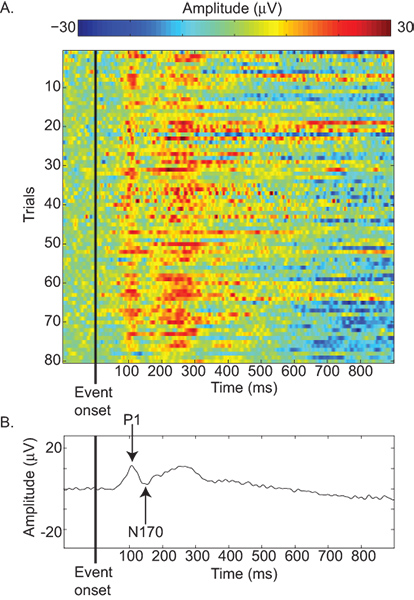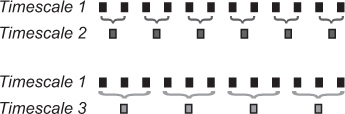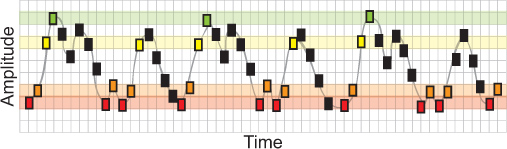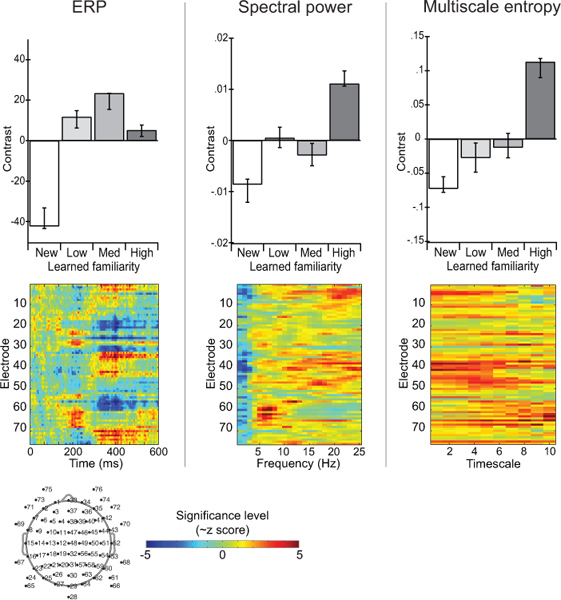Trial ends in

# Applications of EEG Neuroimaging Data: Event-related Potentials, Spectral Power, and Multiscale Entropy

Published: June 27, 2013 doi: 10.3791/50131

### Summary

Neuroimaging researchers typically consider the brain's response as the mean activity across repeated experimental trials and disregard signal variability over time as "noise". However, it is becoming clear that there is signal in that noise. This article describes the novel method of multiscale entropy for quantifying brain signal variability in the time domain.

### Abstract

When considering human neuroimaging data, an appreciation of signal variability represents a fundamental innovation in the way we think about brain signal. Typically, researchers represent the brain's response as the mean across repeated experimental trials and disregard signal fluctuations over time as "noise". However, it is becoming clear that brain signal variability conveys meaningful functional information about neural network dynamics. This article describes the novel method of multiscale entropy (MSE) for quantifying brain signal variability. MSE may be particularly informative of neural network dynamics because it shows timescale dependence and sensitivity to linear and nonlinear dynamics in the data.

### Introduction

Recent advances in neuroimaging have dramatically augmented our understanding of brain function. However, many of the applications of neuroimaging data tend to reinforce the view of the brain in static states rather than emphasizing cognitive operations as they unfold in real time. Consequently, little is known about the space-time structure of brain networks and how the sequence of changes in spatiotemporal patterns across multiple timescales contributes to a specific cognitive operation. The present article describes multiscale entropy (MSE) 5, a new analytic tool for neuroimaging data that examines the complexity of the spatiotemporal pattern underlying specific cognition operations by providing information about how different neural generators in a functional brain network communicate across multiple timescales.

Derived from information theory, an applied branch of mathematics 7,16, MSE was originally designed to examine the complexity of electrocardiograms 4. In theory, MSE could be used to analyze the complexity of any time series; the primary requisite is that the signal time series contains at least 50 data points of continuous time. However, the timescale dependence and sensitivity to linear and nonlinear dynamics in the data may make MSE particularly informative of neural network dynamics.

Here, we focus on the application of MSE to electroencephalogram (EEG) neuroimaging data 9,12. EEG is a noninvasive neuroimaging technique whereby electrodes that are placed on the scalp capture the postsynaptic responses of populations of neurons in neocortex 1. With high temporal resolution, EEG easily meets the time series length requisite of MSE without altering the typical acquisition protocol. To emphasize the utility of the application of MSE to EEG data, we compare this novel method with more traditional approaches including event-related potential and spectral power. When used together, these complementary methods of analysis provide a more complete description of the data that may lead to further insight into neural network operations that give rise to cognition.

### Protocol

1. EEG Acquisition

1. Explain the experimental procedures to the participant and obtain informed consent.
2. Apply drop-down electrodes. Clean area on the face where drop-down electrodes will be located using an alcohol swab.
3. Place electrode cap on the participant's head. Measure participant's head circumference and choose the appropriate cap size. Following the internationally recognized 10-20 system for electrode placement, measure the distance from nasion to inion along the midline and divide by 10%. Using that number, measure up from nasion and mark. Align the electrode cap position Fp with this mark and pull the cap back. Make sure that the center of the cap is in line with the nose. Measure nasion to Cz, and confirm that this distance is half the distance from nasion to inion. Tighten the chinstrap.
4. Place gel-filled blunt-point syringe in the electrode holders. To create a conductive column of gel, start in contact with the scalp, then squeeze and pull back. Note that the application of too much gel may bridge the signals of neighboring electrodes.
5. Fix active electrodes into the electrode holders.
6. Position the subject in front of the monitor at the appropriate distance for the experiment. Ask the participant to remain still, emphasizing the importance of minimizing eye movements and blinks for a clean recording.
7. Examine the electrode connections and EEG signal quality on the acquisition computer. Verify that all electrode offsets are low (< 40 mV) and stable. If there is a problem with a particular electrode, take out that electrode and reapply gel to adjust impedances at that site.
8. Save the file and start the experiment.

2. EEG Analysis

1. After experimentation, but before extracting the particular statistic of interest, preprocess the continuous EEG data to remove artifacts using standard procedures of filtering and artifact rejection. Cut the continuous EEG into epochs corresponding to each discrete event, such as the presentation of photograph. In each epoch, include a 100 msec pre-stimulus window as a baseline.
2. Event-related potentials (ERP) analysis captures synchronous brain activity that is phase-locked to the onset of the event. Average across trials to separate the evoked responses from the "noisy" (i.e. non-phase locked) background activity. The variability across trials and between-subjects presents a major challenge for the ERP method of analysis. To achieve a good signal-to-noise ratio the experimental protocol should include many discrete events with definable onsets. Time locking the brain's response to the onset of a salient event and then averaging over many like events helps to reduce some of this noise; however, the temporal synchrony created by this procedure typically dissolves within 1 sec. Identify ERP component peak amplitudes and latencies for each subject (for more detailed guidelines on ERP analysis, see Picton et al., 2000).
3. Using Fourier analysis, transform the EEG signal from the time domain to the frequency domain and decompose the signal into its composite sine waves of varying frequencies 6.
4. Multiscale entropy (MSE) is an information theoretic metric that estimates the variability of the neuroelectical signals over time and across multiple timescales. To provide a conceptual depiction of MSE analysis, consider two simulated waveforms, a regular waveform and a more stochastic one. Sample entropy values are near zero for the regular waveform and ~2.5 for the more variable waveform. An increase in sample entropy corresponds to an increase in signal complexity, which, according to information theory, can be interpreted as an increase in the amount of information processing capacity of the underlying system 7,16. Remember that the capacity of a brain is not fixed but changes depending on the neural context 2, i.e. the brain regions that happen to be functionally connected at a particular point in time.
5. To calculate MSE, use the algorithm available at www.physionet.org/physiotools/mse/, which computes MSE in two steps.
6. First, the algorithm progressively down-samples the EEG time series per trial and per condition. Down-sample the original time series to generate multiple time series of varying timescales. Time series 1 is the original time series. To create the time series of subsequent timescales, divide the original time series into non-overlapping windows of the timescale length and average the data points within each window. Down-sampling is similar to low-pass filtering; dividing the sampling frequency by the timescale will approximate the frequency at which the signal is low-pass filtered for that particular timescale. The application of MSE to a particular frequency range (e.g., alpha: 9 Hz to 12 Hz) can be interpreted as representing the composition of rhythms within that range as well as the interaction between those frequencies.
7. Second, the algorithm calculates the sample entropy for each coarse-grained time series 14. Sample entropy estimates the complexity of a time series. In a nonlinear analysis of EEG, one assumes that an individual time series represents the manifestation of an underlying multi-dimensional non-linear dynamic model (see Stam, 2005 for a review). In this example, m (the pattern length) is set to two, which means that the variability of the amplitude pattern of each time series will be represented in two-dimensional versus three-dimensional space by considering the sequence pattern of two versus three consecutive data points, respectively. Parameter r (the similarity criterion), reflects the amplitude range (denoted by the height of the colored bands) within which data points are considered to "match". For a typical EEG time series with more than 100 data points, set the parameter m equal to 2 and the parameter r equal to a value between 0.5 and 1 (see Richman and Moorman, 2000; for a detailed procedure on selecting parameters refer to Lake et al., 2002).
To calculate sample entropy for this simulated time series, begin with the first two-component sequence pattern, red-orange. First, count the number of times the red-orange sequence pattern occurs in the time series; there are 10 matches for this two-component sequence. Second, count the number of times first three-component sequence pattern, red-orange-yellow, occurs in the time series; there are 5 matches for this three-component sequence. Continue with the same operations for the next two-component sequence (orange-yellow) and the next three-component sequence (orange-yellow-green) of the time series. The number of two-component matches (5) and three-component matches (3) for these sequences are added to the previous values (total two-component matches = 15; total three-component matches = 8). Repeat for all other sequence matches in the time series (up to N - m) to determine the total ratio of two-component matches to three-component matches. Sample entropy is the natural logarithm of this ratio. For each subject, compute the channel specific MSE estimate as the mean across single trial entropy measures for each timescale.

### Representative Results

Figures 1A and 2A represent the EEG signal in response to the presentation of a face image. Averaging across like trials produces an ERP waveform that consists of a series of positive and negative deflections called ERP components. Figure 1B illustrates an averaged waveform for a single subject and Figure 6A illustrates a grand average waveform for a group of subjects. There is a rich literature that relates each ERP component to a specific perceptual, motor, or cognitive operation. For example, the N170 is a negative deflection that peaks at approximately 170 msec post-stimulus onset and is implicated in face processing 8,15.

Figure 2B illustrates the decomposition of that same EEG signal into component frequency bands. The results from spectral power analysis reveal the frequency content of the signal (Figure 2C), whereby an increase in power at a particular frequency reflects an increase in the presence of that rhythm within the EEG signal.

Like spectral power, MSE is sensitive to the complexity of the oscillatory components contributing to the signal. However, unlike spectral power, MSE is also sensitive to the interactions between frequency components (i.e. nonlinear dynamics 18). The complexity of an EEG signal is represented as a function of sample entropy (Figure 5) over multiple timescales (Figure 4). As illustrated in Figure 3, sample entropy is low for regular signals and increases with the degree of signal randomness. Unlike traditional entropy measures that increase with degree of randomness, multiscale entropy is able to differentiate complex signals from white noise by considering entropy across multiple timescales. For example, Costa et al., 2005 compared multiscale entropy values for uncorrelated (white) noise versus correlated (pink) noise. While sample entropy was greater for white noise than pink noise at fine timescale, the opposite was observed at coarser timescales 5-20. In other words, when entropy was considered across multiple timescales, the true complexity of the signals was more accurately represented than would be if only a single timescale was considered. Depending of the temporal dynamics of a specific contrast, condition effects may be expressed: 1) in the same way across all timescales, 2) at some timescales but not other, or 3) as crossover effects whereby the contrast is different at fine versus coarser timescales.

Figure 6 depicts condition differences in ERP (Figure 6A), spectral power (Figure 6B), MSE (Figure 6C) contrasting the initial versus repeat presentations of face photographs 9. In this example all measures converged to reveal the same effect; however, the observed decrease in sample entropy that accompanies face repetition is important as it constrains the interpretation of the results. A decrease in complexity suggests that the underlying functional network is simpler and capable of processing less information.

Figure 7 depicts statistical results from the multivariate analysis of partial-least squares 11 applied to ERP, spectral power and MSE. The experiment manipulated the familiarity associated with different faces (Heisz et al., 2012). The contrast (bar graph) shows that ERP amplitude distinguished new faces from familiar faces but not among the familiar faces that varied in amount of prior exposure. Spectral power distinguished faces according to acquired familiarity but did not accurately distinguish between the faces of medium and low familiarity. MSE was most sensitive to the condition differences in that sample entropy values increased with increasing face familiarity. The image plots capture the spatiotemporal distribution of the condition effect across all electrodes and time/frequency/timescale. This example demonstrates a situation in which the analysis of EEG by MSE produced unique information that was not obtained using traditional methods of ERP or spectral power. This divergence of MSE suggests that the conditions differ with respect to nonlinear aspects of their network dynamics, possibly involving the interactions between various frequency components.Figure 1. A) The EEG responses of a single subject as a function of amplitude deflection from baseline for each trial plotted against time from the onset of the trial. Each trial consisted of the presentation of a photograph of a face image. Positive amplitude deflections are depicted in red; negative amplitude deflections are depicted in blue. All trials show a positive deflection around 100 msec and 250 msec, indicating event-related phase-locked activity. B) Averaging across all trials depicted in Figure 1A produces an averaged ERP waveform with distinct positive and negative deflections called event-related components and named according to a standard nomenclature. For example, P1 is the first positive going component, and N170 is a negative component that peaks at approximately 170 msec post-stimulus onset.Figure 2. A) The EEG response of a single subject for a single trial plotting amplitude by time (in data points, sampling rate 512 Hz). B) The EEG response of Figure 2A bandpass filtered to isolate frequency bands of delta (0-4 Hz), theta (5-8 Hz), alpha (9-12 Hz), beta (13-30 Hz) and gamma (> 30 Hz). C) Spectral power density of the EEG response depicted in Figure 2A representing frequency composition of the signal as a function of power by frequency. An increase in spectral power at a particular frequency reflects an increase in the number of synchronously active neurons entrained within that particular frequency band. Click here to view larger figure.Figure 3. A) Two simulated waveforms: a regular or predictable waveform depicted in purple, and a more stochastic waveform depicted in black. B) Sample entropy values of the two simulated waveforms for the first three timescales. Sample entropy is low for highly predictable signals than more stochastic signals. Click here to view larger figure.Figure 4. Down-sampling the original time series generates multiple time series of varying timescales. Timescale 1 is the original time series. The time series of timescale 2 is created by dividing the original time series into non-overlapping windows of length 2 and average the data points within each window. To generate the time series of subsequent timescales, divide the original time series into non-overlapping windows of the timescale length and average the data points within each window.Figure 5. A simulated waveform where each rectangle represents a single data point in the time series. Sample entropy estimates the variability of a time series. In this example, m (the pattern length) is set to two, which means that the variance of the amplitude pattern of each time series will be represented in two-dimensional versus three-dimensional space by considering the sequence pattern of two versus three consecutive data points, respectively; r (the similarity criterion), reflects the amplitude range (denoted by the height of the colored bands) within which data points are considered to "match". To calculate sample entropy for this simulated time series, begin with the first two-component sequence pattern, red-orange. First, count the number of times the red-orange sequence pattern occurs in the time series; there are 10 matches for this two-component sequence. Second, count the number of times first three-component sequence pattern, red-orange-yellow, occurs in the time series; there are 5 matches for this three-component sequence. Continue in this manner for the next two-component sequence (orange-yellow) and three-component sequence (orange-yellow-green). The number of two-component matches (5) and three-component matches (3) for these sequences are added to the previous values (total two-component matches =15; total three-component matches = 8). Repeat for all other sequence matches in the time series (up to N - m) to determine the total ratio of two-component matches to three-component matches. Sample entropy is the natural logarithm of this ratio. For each subject, compute the channel specific MSE estimate as the mean across single trial entropy measures for each timescale.Figure 6. Condition differences in ERP (A), spectral power (B), MSE (C) contrasting the initial versus repeated presentations of facial photographs. Click here to view larger figure.Figure 7. Contrasting the EEG response to learned faces across measures of ERP, spectral power, and multiscale entropy. The bar graphs depict the contrast between conditions as determined by partial least squares analysis 11. The image plot highlights the spatial-temporal distribution at which this contrast was most stable as determined by bootstrapping. Values represent ~z scores and negative values denote significance for the inverse condition effect. Click here to view larger figure.

### Discussion

The goal of the present article was to provide a conceptual and methodological description of multiscale entropy (MSE) as it applies to EEG neuroimaging data. EEG is a powerful non-invasive neuroimaging technique that measures neural network activity with the high temporal resolution. The EEG signal reflects post-synaptic activity of populations of pyramidal cells in the cortex, whose collective responses are modified by various excitatory and inhibitory reentrant connections. Accordingly, there are multiple ways to analyze EEG data and each method extracts a unique aspect of the data.

We discussed two common methods of analysis: event-related potential (ERP) analysis and spectral power analysis. ERP analysis captures the synchronous neuronal activity in the EEG signal that is phase-locked to the onset of a discrete event. ERPs reflect specific perceptual, motor, or cognitive operations, making this statistic ideal for examining specific processing stages. Spectral power analysis quantifies the relative contribution of a particular frequency to the EEG signal. Various excitatory and inhibitory feedback loops interact to entrain the activity of neuronal populations at a particular frequency 1,3. Such synchrony between disparate brain regions is thought to promote the binding of information across widespread neural networks. There is a rich literature supporting the link between the power within a particular frequency range and a specific emotional or cognitive state of function 3.

When analyzing EEG it is also important to keep in mind that neural networks are complex systems with non-linear dynamics. Such complexity is reflected in the EEG signal as irregular oscillations that are not the consequence of meaningless background noise. Like synchronous oscillatory activity, the interactions between various excitatory and inhibitory reentrant loops cause transient fluctuations in the brain signal over time 6. Such transients are believed to reflect transitions or bifurcations between network microstates that can be used to estimate the degrees of freedom or complexity of the underlying network; greater variability in the amplitude pattern of the signal over time is indicative of a more complex system 5. Critically, ERP or spectral power analyses are not sensitive to such irregular activity, whereas MSE is. Moreover, an index of network complexity cannot be obtained by simply counting the number of active brain regions as such a method is blind to the transient and dynamic recurrent interactions between brain regions.

Complementary methods for neuroimaging analysis combine to create a complete picture of the underlying neural activity. The interpretation of results from more traditional applications of neuroimaging data, such as ERP and spectral power, are augmented by measures of complexity like MSE; MSE provides a way to capture the sequence of changes in the spatiotemporal patterns of brain activity across multiple timescales that contributes to a specific cognitive operation. Applying MSE to new and existing data sets may provide further insight into how cognition emerges from neural network dynamics.

### Disclosures

No conflicts of interest declared.

### Materials

 Name Company Catalog Number Comments EEG BioSemiPlay Video
DOIX